# Morse alphabet

Calculate how many words of Morse code to create compiling commas and dots in the words of one to four characters.

Result

n =  30

#### Solution:Leave us a comment of example and its solution (i.e. if it is still somewhat unclear...):

Showing 0 comments:Be the first to comment!#### To solve this example are needed these knowledge from mathematics:

See also our variations calculator. Would you like to compute count of combinations?

## Next similar examples:

1. Bits, bytes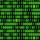Calculate how many different numbers can be encoded in 16-bit binary word?
2. PIN - codesHow many five-digit PIN - code can we create using the even numbers?
3. Area codes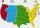How many 6 digit area codes are possible if the first number can't be zero?
4. Olympics metalsIn how many ways can be win six athletes medal positions in the Olympics? Metal color matters.
5. Football league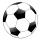In the 5th football league is 10 teams. How many ways can be filled first, second and third place?
6. MedalsIn how many ways can be divided gold, silver and bronze medal among 21 contestant?
7. Cars plates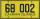How many different licence plates can country have, given that they use 3 letters followed by 3 digits?
8. 7 heroes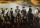9 heroes galloping on 9 horses behind. How many ways can sort them behind?
9. MetalsIn the Hockey World Cup play eight teams, determine how many ways can they win gold, silver and bronze medals.
10. Election 4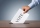In a certain election there are 3 candidates for president 5 for secretory and 2 for tresurer. Find how many ways the election may (turn out/held).
11. Neighborhood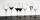I have 7 cups: 1 2 3 4 5 6 7. How many opportunities of standings cups are there if 1 and 2 are always neighborhood?
12. Peak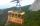Uphill leads 2 paths and 1 lift. a) How many options back and forth are there? b) How many options to get there and back by not same path are there? c) How many options back and forth are there that we go at least once a lift?
13. Combinatorics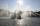The city has 7 fountains. Works only 6. How many options are there that can squirt ?
14. Mumbai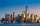A job placement agency in Mumbai had to send ten students to five companies two to each. Two of the companies are in Mumbai and others are outside. Two of the students prefer to work in Mumbai while three prefer to work outside. In how many ways assignment
15. VariationsDetermine the number of items when the count of variations of fourth class without repeating is 42 times larger than the count of variations of third class without repetition.
16. Task of the year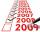Determine the number of integers from 1 to 106 with ending four digits 2006.
17. Theorem proveWe want to prove the sentense: If the natural number n is divisible by six, then n is divisible by three. From what assumption we started?